## Reuse Simulink Functions in Stateflow Charts

A Simulink® function is a graphical object that enables you to call a Simulink subsystem in the actions of states and transitions. Simulink functions are not supported in standalone Stateflow® charts in MATLAB®.

• Defining a function that requires Simulink blocks

• Scheduling execution of multiple controllers

• No function-call subsystem blocks

• No output events

• No signal lines

A Simulink function can reside anywhere in a chart, state, or subchart. The location of a function determines what states and transitions are able to call the function.

• If you want to call the function within only one state or subchart and its substates, put your Simulink function in that state or subchart. That function overrides any other functions of the same name in the parents of that state or subchart.

• If you want to call the function from anywhere in a chart, put your Simulink function at the chart level.

This Simulink function has the name `sim_fcn`. It takes three arguments (`a`, `b`, and `c`) and returns two output values (`x` and `y`).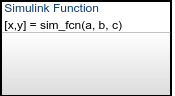The function contains a Simulink subsystem that multiplies the first argument times a gain of 25 and combines the other two arguments into a complex output signal.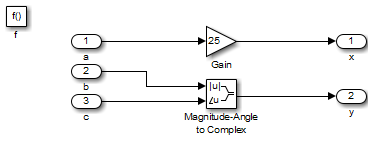Once you have your Simulink function defined, you can place it anywhere in your Stateflow chart or Simulink model. Additionally, you can reuse this function as many times as needed within the same or different models.

Note

You are unable to call global Simulink Functions from a Stateflow chart within a Model Reference. To resolve this issue, create a new Simulink Function in your Stateflow chart. Inside this Simulink Function, include a Function Caller block that calls the original global Simulink Function. For more information on the Function Caller block, see Function Caller (Simulink).

1. In the object palette, click the Simulink function icon.

2. On the chart canvas, click the location for the new Simulink function.

3. Enter the signature label for the function.

The signature label of the function specifies a name for your function and the formal names for its arguments and return values. A signature label has this syntax:

``[return_val1,return_val2,...] = function_name(arg1,arg2,...)``
You can specify multiple return values and multiple input arguments. Each return value and input argument can be a scalar, vector, or matrix of values. For functions with only one return value, omit the brackets in the signature label.

You must use unique variable names for all arguments and return values.

4. To program the function, open the Simulink Editor by double-clicking the function box. Initially, the editor contains a function-call Trigger (Simulink) block and Inport (Simulink) and Outport (Simulink) blocks that match the function signature. You cannot delete the Trigger block.

6. Configure the Inport and Outport blocks.

1. Double-click each block to open the Block Parameters dialog box.

2. In the Signal Attributes tab, enter the Data type and Port dimensions of the input parameter or return value.

3. Click .

Note

An Inport block in a Simulink function cannot inherit its Data type and Port dimensions. For more information, see Guidelines for Using Simulink Functions.

### Call Simulink Functions in States and Transitions

You can call Simulink functions from the actions of any state or transition or from other functions.

To call a Simulink function, use the function signature and include an argument value for each formal argument in the function signature.

``[return_val1,return_val2,...] = function_name(arg1,arg2,...)``

If the data types of the two arguments differ, the function casts the argument to the type of the formal argument.

### Specify Properties of Simulink Functions

You can modify properties for a Simulink function in the Block Parameters dialog box.

1. In the Stateflow Editor, right-click the Simulink function.

2. Select Properties.

3. Edit the Simulink function properties.

You can also modify these properties in the Model Explorer. For more information, see Model Explorer (Simulink).

You can specify additional properties of Simulink functions programmatically by using `Stateflow.SLFunction` objects. For more information about the Stateflow programmatic interface, see Overview of the Stateflow API.

In this example, you can compare modeling the shift logic of a car system. The first model does not use Simulink functions while the second does.

#### Model Without a Simulink Function

This model uses a function-call subsystem, `run()`, Simulink model to calculate the threshold for shifting gears. The Stateflow chart then uses an output event, `CALC_TH()`, to call the subsystem.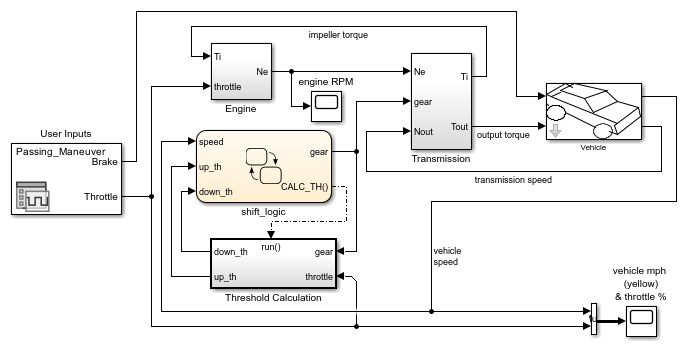#### Model With a Simulink Function

This same functionality can be achieved with a Simulink function. In this Stateflow chart, the Simulink function `calc_th` is used to calculate the threshold.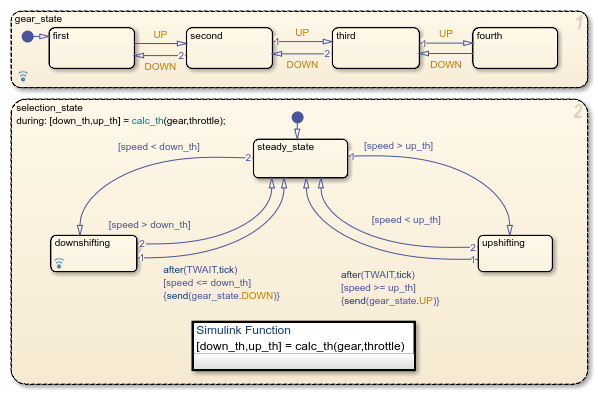The `during` action in `selection_state` contains a function call to `calc_th`, which contains Simulink blocks.

This modeling method minimizes the objects in your model.### Use a Simulink Function to Schedule Execution of Multiple Controllers

In this example, you can compare two ways of scheduling execution of multiple controllers. The first model does not use Simulink functions while the second does.

This model uses function-call subsystems to model each controller. The model includes output events in a Stateflow chart to schedule execution of the subsystems.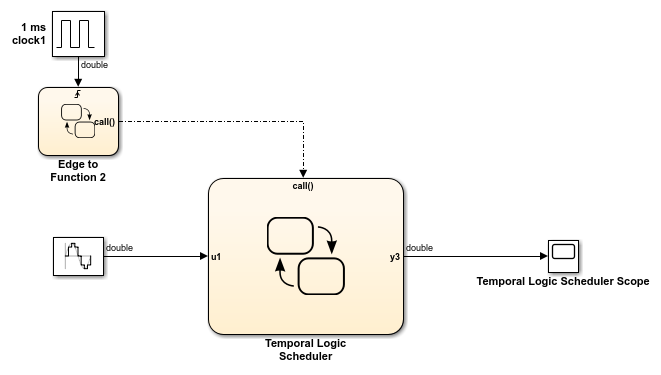For each output event, a signal line is needed to connect the Stateflow chart with the corresponding function-call subsystem.

#### Model Method With Simulink Functions

Each controller can also be modeled with a Simulink function in a Stateflow chart. This model uses function calls to schedule execution of the subsystems.This modeling method minimizes the objects in your model.